Quality and Process Methods > Legacy Control Charts > Statistical Details for Legacy Control Charts > Statistical Details for Weighted Moving Average Charts
Publication date: 09/27/2021

## Statistical Details for Weighted Moving Average Charts

This section describes how control limits are calculated in legacy weighted moving average charts.

### Control Limits for UWMA Charts

Control limits for UWMA charts are computed for each subgroup i as follows:

LCLi =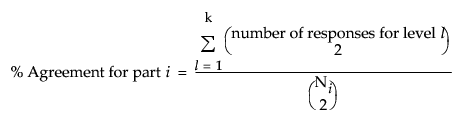UCLi =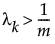where:

w is the span parameter (number of terms in moving average)

ni is the sample size of the ith subgroup

k is the number of standard deviations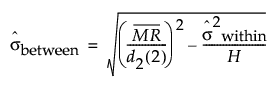is the weighted average of the subgroup means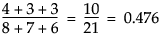is the estimated process standard deviation

### Control Limits for EWMA Charts

Control limits for EWMA charts are computed as follows:

LCL =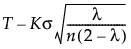UCL =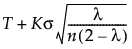where:

r is the EWMA weight parameter (0 < r 1)

xij is the jth measurement in the ith subgroup, with j = 1, 2, 3,..., ni

ni is the sample size of the ith subgroup

k is the number of standard deviations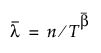is the weighted average of the subgroup meansis the estimated process standard deviation